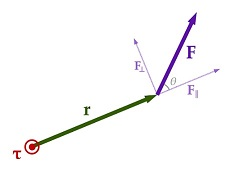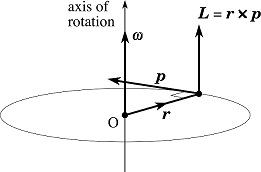# System of Particles and Rotational Motion - Online Test

Q1. The moment of inertia of a body about any axis is
Explaination / Solution:

according to parallel axis theorem the moment of inertia of a body about any axis is equal to the sum of the moment of inertia of the body about a parallel axis passing through its Centre of mass and the product of its mass and the square of the distance between the two parallel axes.

Q2. Angular acceleration vector is defined as
Explaination / Solution:

As per the definition of angular accerlation  that " it is the time rate of change of  angular velocity" , therefore
Q3. Torque τ is defined in terms of position vector r and the force acting F as
Explaination / Solution:

Torque = Force x Perpendicular distance of the line of action of the force from the axis of rotation. It is also called moment of force.

torque is defined as the cross product of the vector by which the force's application point is offset relative to the fixed suspension point (distance vector) and the force vector, which tends to produce rotational motion.Q4. The angular momentum L of the particle having a position vector r and linear momentum p, with respect to the origin O is defined to be
Explaination / Solution:

Angular momentum is also referred as moment of linear momentum

The angular momentum L of a particle of mass m with respect to a chosen origin is given by

Direction of angular momentum is according to right hand rule.Q5. Centre of gravity can be defined
Explaination / Solution:

The point at which the entire weight of a body may be thought of as centered so that if supported at this point the body would balance perfectly, so it can also be defined as as that point where the total gravitational torque on the body is zero.

Q6. Analogue of mass in rotational motion is
Explaination / Solution:

The moment of inertia is a physical quantity which tells how easily a body can be rotated about a given axis. It is a rotational analogue of mass. It plays the same role in rotational motion as 'inertia' does in translational motion. Large moment of inertia also helps in keeping the rotation motion uniform.

Q7. The radius of gyration of a body about an axis may be defined as the
Explaination / Solution:

It is the perpendicular distance from the axis of rotation to a point mass of mass m that gives an equivalent inertia to the original object (of mass, m)

if radius of gyration is k then

Q8. The moment of inertia of a planar body (lamina) about an axis perpendicular to its plane is.
Explaination / Solution:

According to perpendicular axis  theorem "For a planar object, the moment of inertia about an axis perpendicular to the plane is the sum of the moments of inertia of two perpendicular axes through the same point in the plane of the object".

Q9. The moment of inertia of a body about any axis is
Explaination / Solution:

according to parallel axis theorem the moment of inertia of a body about any axis is equal to the sum of the moment of inertia of the body about a parallel axis passing through its Centre of mass and the product of its mass and the square of the distance between the two parallel axes.

Q10. For rolling motion without slipping the kinetic energy of the body is
Explaination / Solution:

Rolling motion without slipping involve both translation motion and rotation motion. Hence kinetic energy of body in rolling motion is the  sum of both translation kinetic energy and rotational kinetic energy.